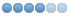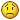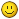# Step/Dir servos + Encoders = follow errors... and so much pain.....

• jhandel
• Offline
•21 Dec 2020 04:29 - 21 Dec 2020 04:31 #192608 by jhandel
Ok,
I have burned I don't know how much time trying to tune my servos... As it stands they home fine, some slow stuff is fine.. but like if I set my MPG at 100x (100 pulses) then I get a follow error off the first tick... I can do some rapids but to many back and forths... get a follow error.. Really all I need is the ability to home off the Z-pulse.. I am happy to ignore the encoders the rest of the time... However, (as I learned a few months back) you can't mix Z-pulse (HOME_USE_INDEX) and stepgen.position-fb..

I am all ears on some process to tune step/dir based servos with encoders that can get these things going OR a work around so that I can use HOME_USE_INDEX + stepgen.position-fb...

Here is my current INI (with PID settings) for reference...
``` Generated by PNCconf at Sat Oct  3 17:03:13 2020
# Using LinuxCNC version:  UNAVAILABLE
# If you make changes to this file, they will be
# overwritten when you run PNCconf again

[EMC]
MACHINE = Skyfire
DEBUG = 0
VERSION = 1.1

[DISPLAY]
DISPLAY = probe_basic
CONFIRM_EXIT = False
CONFIG_FILE = custom_config.yml
FULLSCREEN = False
MAXIMIZE = True
POSITION_OFFSET = RELATIVE
POSITION_FEEDBACK = ACTUAL
MAX_FEED_OVERRIDE = 2.000000
MAX_SPINDLE_OVERRIDE = 1.000000
MIN_SPINDLE_OVERRIDE = 0.500000
INTRO_GRAPHIC = linuxcnc.gif
INTRO_TIME = 1
PROGRAM_PREFIX = /home/cnc/linuxcnc/nc_files
INCREMENTS = 5mm 1mm .5mm .1mm .05mm .01mm .001mm
POSITION_FEEDBACK = ACTUAL
DEFAULT_LINEAR_VELOCITY = 6.000000
MAX_LINEAR_VELOCITY = 167.000000
MIN_LINEAR_VELOCITY = 0.500000
DEFAULT_ANGULAR_VELOCITY = 12.000000
MAX_ANGULAR_VELOCITY = 180.000000
MIN_ANGULAR_VELOCITY = 1.666667
EDITOR = gedit
GEOMETRY = xyz

[VTK]
# VTK_Widget Options
MACHINE_BOUNDRY = False
MACHINE_TICKS = False
MACHINE_LABELS = False

PROGRAM_BOUNDRY = False
PROGRAM_TICKS = False
PROGRAM_LABELS = False
GRID_LINES = False

[FILTER]
PROGRAM_EXTENSION = .png,.gif,.jpg Greyscale Depth Image
PROGRAM_EXTENSION = .py Python Script
png = image-to-gcode
gif = image-to-gcode
jpg = image-to-gcode
py = python

CYCLE_TIME = 0.010

[ATC]
# Carousel image available for 8, 10, 12, 14, 16, 18, 20, 21, 24
POCKETS = 12

[PYTHON]
TOPLEVEL= /home/cnc/linuxcnc/configs/Skyfire/python/toplevel.py
PATH_APPEND= /home/cnc/linuxcnc/configs/Skyfire/python/
PATH_APPEND= /home/cnc/linuxcnc/configs/Skyfire/python/python-stdglue/

[RS274NGC]
PARAMETER_FILE = linuxcnc.var
RS274NGC_STARTUP_CODE = F10 S300 G21 G17 G40 G49 G54 G64 P0.001 G80 G90 G91.1 G92.1 G94 G97 G98 M22
FEATURES = 30
SUBROUTINE_PATH = /home/cnc/linuxcnc/configs/Skyfire/subroutines
REMAP=M6  modalgroup=6 prolog=change_prolog ngc=toolchange epilog=change_epilog
REMAP=M10 modalgroup=6 argspec=p ngc=m10
REMAP=M11 modalgroup=6 argspec=p ngc=m11
REMAP=M12 modalgroup=6 argspec=p ngc=m12
REMAP=M13 modalgroup=6 ngc=m13

REMAP=M21 modalgroup=6 ngc=m21
REMAP=M22 modalgroup=6 ngc=m22
REMAP=M23 modalgroup=6 ngc=m23
REMAP=M24 modalgroup=6 ngc=m24

[EMCMOT]
EMCMOT = motmod
COMM_TIMEOUT = 1.0
SERVO_PERIOD = 1000000

[HMOT]
# **** This is for info only ****
CARD0=hm2_7i76.0

[HAL]
HALUI = halui
HALFILE = init.hal
HALFILE = io.hal
HALFILE = axis.hal
HALFILE = spindle.hal
HALFILE = mpg.hal
POSTGUI_HALFILE = messages.hal
SHUTDOWN = shutdown.hal

[HALUI]

[KINS]
JOINTS = 3
KINEMATICS = trivkins coordinates=XYZ

[TRAJ]
COORDINATES =  XYZ
LINEAR_UNITS = mm
ANGULAR_UNITS = degree
DEFAULT_LINEAR_VELOCITY = 32.00
MAX_LINEAR_VELOCITY = 600.00
POSITION_FILE = position.txt

[EMCIO]
EMCIO = io
CYCLE_TIME = 0.100
TOOL_TABLE = tool.tbl
TOOL_CHANGE_WITH_SPINDLE_ON = 1
TOOL_CHANGE_QUILL_UP = 1

#******************************************
[AXIS_X]
MAX_VELOCITY = 166.666666667
MAX_ACCELERATION = 225.0
MIN_LIMIT = 0
MAX_LIMIT = 420.0

[JOINT_0]
TYPE = LINEAR
HOME = 210.0
FERROR = 1
MIN_FERROR = 0.05
MAX_VELOCITY = 166.666666667
MAX_ACCELERATION = 225.0
# The values below should be 25% larger than MAX_VELOCITY and MAX_ACCELERATION
# If using BACKLASH compensation STEPGEN_MAXACCEL should be 100% larger.
STEPGEN_MAXVEL = 208.33
STEPGEN_MAXACCEL = 281.25
P = 225.0
I = 0.0
D = 0.0
FF0 = 0.0
FF1 = 1.0
FF2 = 0.0
BIAS = 0.0
MAX_OUTPUT = 0.0
ENCODER_SCALE = 2000.0
# these are in nanoseconds
DIRSETUP   = 1000
DIRHOLD    = 1000
STEPLEN    = 1000
STEPSPACE  = 1000
STEP_SCALE = -1000.0
MIN_LIMIT = 0
MAX_LIMIT = 420.0
HOME_OFFSET = 396.000000
HOME_SEARCH_VEL = 20.000000
HOME_LATCH_VEL = 0.500000
HOME_FINAL_VEL = 50.000000
HOME_USE_INDEX = YES
HOME_SEQUENCE =  1
VOLATILE_HOME = 0
#******************************************

#******************************************
[AXIS_Y]
MAX_VELOCITY = 166.666666667
MAX_ACCELERATION = 225.0
MIN_LIMIT = 0
MAX_LIMIT = 230.0

[JOINT_1]
TYPE = LINEAR
HOME = 115.0
FERROR = 1
MIN_FERROR = 0.05
MAX_VELOCITY = 166.666666667
MAX_ACCELERATION = 225.0
# The values below should be 25% larger than MAX_VELOCITY and MAX_ACCELERATION
# If using BACKLASH compensation STEPGEN_MAXACCEL should be 100% larger.
STEPGEN_MAXVEL = 208.33
STEPGEN_MAXACCEL = 281.25
P = 225.0
I = 0.0
D = 0.0
FF0 = 0.0
FF1 = 1.0
FF2 = 0.0
BIAS = 0.0
MAX_OUTPUT = 0.0
ENCODER_SCALE = 2000.0
# these are in nanoseconds
DIRSETUP   = 1000
DIRHOLD    = 1000
STEPLEN    = 1000
STEPSPACE  = 1000
STEP_SCALE = -1000.0
MIN_LIMIT = 0
MAX_LIMIT = 230.0
HOME_OFFSET = 212.000000
HOME_SEARCH_VEL = 20.000000
HOME_LATCH_VEL = 0.500000
HOME_FINAL_VEL = 50.000000
HOME_USE_INDEX = YES
HOME_SEQUENCE =  -2
VOLATILE_HOME = 0
#******************************************

#******************************************
[AXIS_Z]
MAX_VELOCITY = 166.666666667
MAX_ACCELERATION = 225.0
MIN_LIMIT = -430
MAX_LIMIT = 10.0

[JOINT_2]
TYPE = LINEAR
HOME = -14.0
FERROR = 1
MIN_FERROR = 0.05
MAX_VELOCITY = 166.666666667
MAX_ACCELERATION = 225.0
# The values below should be 25% larger than MAX_VELOCITY and MAX_ACCELERATION
# If using BACKLASH compensation STEPGEN_MAXACCEL should be 100% larger.
STEPGEN_MAXVEL = 208.33
STEPGEN_MAXACCEL = 281.25
P = 150.0
I = 0.0
D = 0.0
FF0 = 0.0
FF1 = 1.0
FF2 = 0.0
BIAS = 0.0
MAX_OUTPUT = 0.0
ENCODER_SCALE = -2000.0
# these are in nanoseconds
DIRSETUP   = 1000
DIRHOLD    = 1000
STEPLEN    = 1000
STEPSPACE  = 1000
STEP_SCALE = 1000.0
MIN_LIMIT = -430
MAX_LIMIT = 10.0
HOME_OFFSET = -7.000000
HOME_SEARCH_VEL = 10.000000
HOME_LATCH_VEL = 0.500000
HOME_FINAL_VEL = 10.000000
HOME_USE_INDEX = YES
HOME_SEQUENCE =  0
VOLATILE_HOME = 0
#******************************************

[SPINDLE_0]
MAX_VELOCITY = 150.0
MAX_ACCELERATION = 30.0
# The values below should be 25% larger than MAX_VELOCITY and MAX_ACCELERATION
# If using BACKLASH compensation STEPGEN_MAXACCEL should be 100% larger.
STEPGEN_MAXVEL = 187.50
STEPGEN_MAXACCEL = 60.00
P = 0.0
I = 0.0
D = 0.0
FF0 = 1.0
FF1 = 0.0
FF2 = 0.0
BIAS = 0.0
MAX_OUTPUT = 9000.0
ENCODER_SCALE = 4096.0
# these are in nanoseconds
DIRSETUP   = 2000
DIRHOLD    = 2000
STEPLEN    = 1000
STEPSPACE  = 1000
STEP_SCALE = 2500.0```

Anyways, you guys are all far smarter than I so, yah, going to ask the smart folks..

(upside, I have quite a bit working really well... E-stop latches, soft start & stop on the spindle, MPG, all the ATC stuff is coming along great.. but man if I can't get my servo's "tuned"...... And most of the tuning instructions don't seem to work all to well with Step/Dir systems...
Last edit: 21 Dec 2020 04:31 by jhandel.

21 Dec 2020 12:07 #192629
Hal file ?
Step/dir servos should be easy to tune, so in general the procedure should be: disconnect the encoder feedback in hal, tune the drives to work properly in step/dir or position mode, then reconnect the feedback in hal and test again. If the drives/motors are set properly, that is all.

• jhandel
• Offline
•21 Dec 2020 13:25 - 21 Dec 2020 13:40 #192639 by jhandel
Tommy,

Thanks for offering the suggestion..

Here is the Hal file for my axises... But before I throw it out.. I will say I have not had that experience..

I can run my servos at P=1000 (advice from PCW a long while back) with feedback off and the machine hums... I can't find a P value that won't cause a follow errors when I start using the encoders.. Granted I have not touched I or D, but everything I have read suggested you start with a stable P value then I and D are bonus point..

That being said, here is the HAL for the axises
```#*******************
#  AXIS X JOINT 0
#*******************

setp   pid.x.Pgain     [JOINT_0]P
setp   pid.x.Igain     [JOINT_0]I
setp   pid.x.Dgain     [JOINT_0]D
setp   pid.x.bias      [JOINT_0]BIAS
setp   pid.x.FF0       [JOINT_0]FF0
setp   pid.x.FF1       [JOINT_0]FF1
setp   pid.x.FF2       [JOINT_0]FF2
setp   pid.x.maxoutput [JOINT_0]MAX_OUTPUT
setp   pid.x.error-previous-target true

net x-index-enable  <=> pid.x.index-enable
net x-enable        =>  pid.x.enable
net x-pos-cmd       =>  pid.x.command
net x-pos-fb        =>  pid.x.feedback
net x-output        <=  pid.x.output

# Step Gen signals/setup

setp   hm2_7i76e.0.stepgen.00.dirsetup        [JOINT_0]DIRSETUP
setp   hm2_7i76e.0.stepgen.00.dirhold         [JOINT_0]DIRHOLD
setp   hm2_7i76e.0.stepgen.00.steplen         [JOINT_0]STEPLEN
setp   hm2_7i76e.0.stepgen.00.stepspace       [JOINT_0]STEPSPACE
setp   hm2_7i76e.0.stepgen.00.position-scale  [JOINT_0]STEP_SCALE
setp   hm2_7i76e.0.stepgen.00.step_type        0
setp   hm2_7i76e.0.stepgen.00.control-type     1
setp   hm2_7i76e.0.stepgen.00.maxaccel         [JOINT_0]STEPGEN_MAXACCEL
setp   hm2_7i76e.0.stepgen.00.maxvel           [JOINT_0]STEPGEN_MAXVEL

# ---closedloop stepper signals---

net x-pos-cmd    <= joint.0.motor-pos-cmd
net x-vel-cmd    <= joint.0.vel-cmd
net x-output     <= hm2_7i76e.0.stepgen.00.velocity-cmd
net x-enable     <= joint.0.amp-enable-out
net x-enable     => hm2_7i76e.0.stepgen.00.enable

# ---Encoder feedback signals/setup---

setp    hm2_7i76e.0.encoder.02.counter-mode 0
setp    hm2_7i76e.0.encoder.02.filter 1
setp    hm2_7i76e.0.encoder.02.index-invert 0
setp    hm2_7i76e.0.encoder.02.scale  [JOINT_0]ENCODER_SCALE

net x-pos-fb               <=  hm2_7i76e.0.encoder.02.position
net x-vel-fb               <=  hm2_7i76e.0.encoder.02.velocity
net x-pos-fb               =>  joint.0.motor-pos-fb
net x-index-enable    joint.0.index-enable  <=>  hm2_7i76e.0.encoder.02.index-enable
net x-pos-rawcounts        <=  hm2_7i76e.0.encoder.02.rawcounts

# ---setup home / limit switch signals---

net home-x     =>  joint.0.home-sw-in

net machine_limit => joint.0.neg-lim-sw-in
net machine_limit => joint.0.pos-lim-sw-in

#*******************
#  AXIS Y JOINT 1
#*******************

setp   pid.y.Pgain     [JOINT_1]P
setp   pid.y.Igain     [JOINT_1]I
setp   pid.y.Dgain     [JOINT_1]D
setp   pid.y.bias      [JOINT_1]BIAS
setp   pid.y.FF0       [JOINT_1]FF0
setp   pid.y.FF1       [JOINT_1]FF1
setp   pid.y.FF2       [JOINT_1]FF2
setp   pid.y.maxoutput [JOINT_1]MAX_OUTPUT
setp   pid.y.error-previous-target true

net y-index-enable  <=> pid.y.index-enable
net y-enable        =>  pid.y.enable
net y-pos-cmd       =>  pid.y.command
net y-pos-fb        =>  pid.y.feedback
net y-output        <=  pid.y.output

# Step Gen signals/setup

setp   hm2_7i76e.0.stepgen.01.dirsetup        [JOINT_1]DIRSETUP
setp   hm2_7i76e.0.stepgen.01.dirhold         [JOINT_1]DIRHOLD
setp   hm2_7i76e.0.stepgen.01.steplen         [JOINT_1]STEPLEN
setp   hm2_7i76e.0.stepgen.01.stepspace       [JOINT_1]STEPSPACE
setp   hm2_7i76e.0.stepgen.01.position-scale  [JOINT_1]STEP_SCALE
setp   hm2_7i76e.0.stepgen.01.step_type        0
setp   hm2_7i76e.0.stepgen.01.control-type     1
setp   hm2_7i76e.0.stepgen.01.maxaccel         [JOINT_1]STEPGEN_MAXACCEL
setp   hm2_7i76e.0.stepgen.01.maxvel           [JOINT_1]STEPGEN_MAXVEL

# ---closedloop stepper signals---

net y-pos-cmd    <= joint.1.motor-pos-cmd
net y-vel-cmd    <= joint.1.vel-cmd
net y-output     <= hm2_7i76e.0.stepgen.01.velocity-cmd
net y-enable     <= joint.1.amp-enable-out
net y-enable     => hm2_7i76e.0.stepgen.01.enable

# ---Encoder feedback signals/setup---

setp    hm2_7i76e.0.encoder.01.counter-mode 0
setp    hm2_7i76e.0.encoder.01.filter 1
setp    hm2_7i76e.0.encoder.01.index-invert 0
setp    hm2_7i76e.0.encoder.01.scale  [JOINT_1]ENCODER_SCALE

net y-pos-fb               <=  hm2_7i76e.0.encoder.01.position
net y-vel-fb               <=  hm2_7i76e.0.encoder.01.velocity
net y-pos-fb               =>  joint.1.motor-pos-fb
net y-index-enable    joint.1.index-enable  <=>  hm2_7i76e.0.encoder.01.index-enable
net y-pos-rawcounts        <=  hm2_7i76e.0.encoder.01.rawcounts

# ---setup home / limit switch signals---

net home-y     =>  joint.1.home-sw-in
net machine_limit => joint.1.neg-lim-sw-in
net machine_limit => joint.1.pos-lim-sw-in

#*******************
#  AXIS Z JOINT 2
#*******************

setp   pid.z.Pgain     [JOINT_2]P
setp   pid.z.Igain     [JOINT_2]I
setp   pid.z.Dgain     [JOINT_2]D
setp   pid.z.bias      [JOINT_2]BIAS
setp   pid.z.FF0       [JOINT_2]FF0
setp   pid.z.FF1       [JOINT_2]FF1
setp   pid.z.FF2       [JOINT_2]FF2
setp   pid.z.maxoutput [JOINT_2]MAX_OUTPUT
setp   pid.z.error-previous-target true

net z-index-enable  <=> pid.z.index-enable
net z-enable        =>  pid.z.enable
net z-pos-cmd       =>  pid.z.command
net z-pos-fb        =>  pid.z.feedback
net z-output        <=  pid.z.output

# Step Gen signals/setup

setp   hm2_7i76e.0.stepgen.02.dirsetup        [JOINT_2]DIRSETUP
setp   hm2_7i76e.0.stepgen.02.dirhold         [JOINT_2]DIRHOLD
setp   hm2_7i76e.0.stepgen.02.steplen         [JOINT_2]STEPLEN
setp   hm2_7i76e.0.stepgen.02.stepspace       [JOINT_2]STEPSPACE
setp   hm2_7i76e.0.stepgen.02.position-scale  [JOINT_2]STEP_SCALE
setp   hm2_7i76e.0.stepgen.02.step_type        0
setp   hm2_7i76e.0.stepgen.02.control-type     1
setp   hm2_7i76e.0.stepgen.02.maxaccel         [JOINT_2]STEPGEN_MAXACCEL
setp   hm2_7i76e.0.stepgen.02.maxvel           [JOINT_2]STEPGEN_MAXVEL

# ---closedloop stepper signals---

net z-pos-cmd    <= joint.2.motor-pos-cmd
net z-vel-cmd    <= joint.2.vel-cmd
net z-output     <= hm2_7i76e.0.stepgen.02.velocity-cmd
net z-enable     <= joint.2.amp-enable-out
net z-enable     => hm2_7i76e.0.stepgen.02.enable

# ---Encoder feedback signals/setup---

setp    hm2_7i76e.0.encoder.00.counter-mode 0
setp    hm2_7i76e.0.encoder.00.filter 1
setp    hm2_7i76e.0.encoder.00.index-invert 0
setp    hm2_7i76e.0.encoder.00.scale  [JOINT_2]ENCODER_SCALE

net z-pos-fb               <=  hm2_7i76e.0.encoder.00.position
net z-vel-fb               <=  hm2_7i76e.0.encoder.00.velocity
net z-pos-fb               =>  joint.2.motor-pos-fb
net z-index-enable    joint.2.index-enable  <=>  hm2_7i76e.0.encoder.00.index-enable
net z-pos-rawcounts        <=  hm2_7i76e.0.encoder.00.rawcounts

# ---setup home / limit switch signals---

net home-z     =>  joint.2.home-sw-in
net machine_limit => joint.2.neg-lim-sw-in
net machine_limit => joint.2.pos-lim-sw-in```

One last note.. These are AC servos being driven by Step/Dir so the servo driver has its own PID control it is running... I can (and with the previous controller did) just "trust' that the servo driver would get the servo where it belonged in time and could just feed it steps... Its only the need for z-pulse based homing that is causing me to try to tune the servos..

(granted, yes if we can get the servo feedback working then of course it will greatly improve general performance.. totally agree with that)

Final, Final notes.. I have cut the acceleration in about 1/2 of what the old controllers would run these servos at... And (at least the Z axis) sounds SUPER rough when running very slow (hunting for z-pulse)

And here is my entire config (I keep it on Github)
github.com/Skyfire-community/SVM2-LinuxCNC

Thanks
Last edit: 21 Dec 2020 13:40 by jhandel.

21 Dec 2020 13:45 #192643
In the hal file:
stepgen 00 is wired to encoder 02
and
stepgen 02 is wired to encoder 00
To check if that is correct, disable the drives and move the motors slowly by hand while watching the DRO, check if the X axis moves the X DRO, etc.

• jhandel
• Offline
•21 Dec 2020 14:23 - 21 Dec 2020 14:24 #192651 by jhandel
These are correct.....

I can make moves with the machine.. Its just that the Follow Error trips ridiculously easily..

For instance, I can run 3dchips sample slowly for about 30% of the file.. If I up the speed to what i know the machine can handle or running at 100ipm like I know the machine can handle because I did it with the old controller... Not a chance..

Thanks though for double checking that is intentional..
Last edit: 21 Dec 2020 14:24 by jhandel.

21 Dec 2020 14:36 #192652 by PCW
Can you widen the following error limits and do some plots of following error
and joint velocity (with halscope)? That's the best way to diagnose what's
going on.

• jhandel
• Offline
•21 Dec 2020 14:41 #192654 by jhandel
What kinds of speeds should I plot at? I'll run a few different plots over lunch..

21 Dec 2020 15:21 #192658 by PCW
i would do a motion at about 1/2 full speed.
Its most important to capture the acceleration/deccelration
sections at maybe 50 ms/division.

• chris@cnc
• Offline
• Platinum Member
•21 Dec 2020 15:35 #192659
And your encoder resolution is real high. Do you really need 0,5µ?
For exmaple i have tuned my servo drive real strong. P in linuxcnc real low to 5. Check with a dial indicator 1mm step. Once with feedback once without. Maybe your counting is not correct. I check this with 1m block. touch one side and drive in EMG by hand to second side and touch. So could see in realitiv coordinates that count your scale correct.
One thing I had too. My encoder cable was of poor quality. So I also had mistakes.

• jhandel
• Offline
•